# Quantum sphere

(diff) ← Older revision | Latest revision (diff) | Newer revision → (diff)

A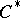-algebragenerated by two elementsandsatisfying [a1]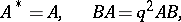Here,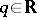is a deformation parameter and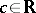is another parameter labeling the family of quantum spheres. Each quantum sphere is a quantum homogeneous space in the sense that there exists a right co-actionwhere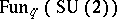stands for the quantum group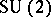[a7] (cf. also Quantum groups) considered as a deformation of the Poisson algebra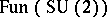. The one-parameter family of quantum spheres is in correspondence with the family of-covariant Poisson structures on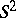, which is known to be one-parametric too ([a2], Appendix). The deformation of the Poisson structure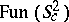can be introduced in a precisely defined manner [a2]. Also, the structure of representations of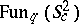is in correspondence with the structure of symplectic leaves on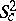[a1], [a2]. For, the symplectic leaves are two open discs and the points of a circle separating them. For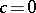, one disc leaf is attached to one one-point leaf and, in fact, this is the Bruhat decomposition of the Poisson homogeneous space. For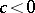,is a symplectic manifold.

The symplectic spheres, with, can be realized as orbits of the dressing transformation ofacting on its dual Poisson Lie group. An equivalent realization is given by the right-action on the manifoldof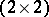unimodular positive matrices, which is just the unitary transformation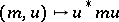. There exists a quantum analogue as a right co-action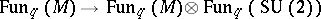, which is defined formally in the same way as in the Poisson case. If,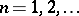, one can construct, using this structure, the-dimensional irreducible representation of the deformed universal enveloping algebra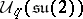[a3]. Moreover, if, then the-algebrais non-trivial only for[a1].

A few other concepts have been developed for quantum spheres, including a description in terms of a local holomorphic coordinateand its adjoint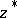[a3], [a4] and a differential and integral calculus [a4], [a5]. In a precise analogy with the classical case, quantum spherical functions were defined as special basis elements inand expressed in terms of big-Jacobi polynomials [a6].

How to Cite This Entry:
Quantum sphere. Encyclopedia of Mathematics. URL: http://encyclopediaofmath.org/index.php?title=Quantum_sphere&oldid=13378
This article was adapted from an original article by Pavel Å tovÃ­Äek (originator), which appeared in Encyclopedia of Mathematics - ISBN 1402006098. See original article